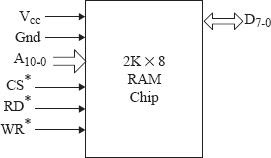Pin details and its address range in 8085 Microprocessor

MicrocontrollerMicroprocessor8085

Let us assume that we have a RAM chip of 2K x 8, It signifies that, we have 2 x 1024 = 2048 memory locations having information of 8 bits at each location. For selecting one of 2K = 211 memory locations in chip, there should be exactly input pins of 11 address lines. For bidirectional data transfer scheme, there should be eight pin involved in this process. The processor action involved in it is signified by the input pins RD* and WR*. Two pins are specified for grounding process of the pin and connecting of Direct Current of +5 VOLT directly to the supplied power.

There is also a pin for selection which is in active low state. Now the question arises to all of us what should be the starting address of the chip, if it starts with 0000H. Often we increase the capacity of the RAM. As a convention the point should be noted that, the range of memory address which is added must not overlap with the address range which was existing. A computer designer puts the memory like his own way to the range of address. But in 8085 the range of address should be 0000H-FFFFH. We know that we have address input pins of 11 to select the memory locations of 2K = 211. It is quite simple and easy for us to see that C000H-C7FFH has actually memory allocations and specified locations of 2K. We have all total four locations in all namely C000H, C001H, C002H, and C003H. Simplified way if the range of address of memory extends from X to Y, the sum total of memory allocated is (Y - X) + 1. So the total number of located memory in the range of address is from C000H to C7FFH which is 7FFH + 1 = 800H i.e. 2048 = 2K.Fig: Diagram of functional pin of 2k x 8 RAM chip

The circuit below gives us information to know the range of ranging from C000H–C7FFH.A15-11 selects RAM
A10-0 selects a location in RAM
1 1 0 0 0
0 0 0 0 0 0 0 0 0 0 0 = C000H

Fig: Equivalent circuit of RAM selection